# 6.4: The multiplier - Changes in aggregate expenditure and equilibrium output

$$\newcommand{\vecs}{\overset { \rightharpoonup} {\mathbf{#1}} }$$ $$\newcommand{\vecd}{\overset{-\!-\!\rightharpoonup}{\vphantom{a}\smash {#1}}}$$$$\newcommand{\id}{\mathrm{id}}$$ $$\newcommand{\Span}{\mathrm{span}}$$ $$\newcommand{\kernel}{\mathrm{null}\,}$$ $$\newcommand{\range}{\mathrm{range}\,}$$ $$\newcommand{\RealPart}{\mathrm{Re}}$$ $$\newcommand{\ImaginaryPart}{\mathrm{Im}}$$ $$\newcommand{\Argument}{\mathrm{Arg}}$$ $$\newcommand{\norm}{\| #1 \|}$$ $$\newcommand{\inner}{\langle #1, #2 \rangle}$$ $$\newcommand{\Span}{\mathrm{span}}$$ $$\newcommand{\id}{\mathrm{id}}$$ $$\newcommand{\Span}{\mathrm{span}}$$ $$\newcommand{\kernel}{\mathrm{null}\,}$$ $$\newcommand{\range}{\mathrm{range}\,}$$ $$\newcommand{\RealPart}{\mathrm{Re}}$$ $$\newcommand{\ImaginaryPart}{\mathrm{Im}}$$ $$\newcommand{\Argument}{\mathrm{Arg}}$$ $$\newcommand{\norm}{\| #1 \|}$$ $$\newcommand{\inner}{\langle #1, #2 \rangle}$$ $$\newcommand{\Span}{\mathrm{span}}$$$$\newcommand{\AA}{\unicode[.8,0]{x212B}}$$

In our model the slope of the AE line depends on the marginal propensity to consume and the marginal propensity to import. For any given mpc and mpm, the level of autonomous expenditure (A0) determines the height of the AE line. Recall that autonomous expenditure is expenditure that is not related to national income.

In a diagram, changes in autonomous expenditure cause parallel vertical shifts in the AE function. Autonomous expenditure depends chiefly on current expectations about future domestic and foreign demand for output, future prices and future profits. These expectations about the size and strength of future markets can fluctuate significantly, influenced by current pessimism or optimism about the future. We saw this volatility in investment in Figure 6.6. Similarly, changes in conditions in export markets change exports and changes in consumer confidence change autonomous consumption expenditure.

Suppose firms become very optimistic about future demand for their output. They want to expand their factories and add new equipment to meet this future demand. Autonomous expenditure rises. If other components of aggregate expenditure are unaffected, AE will be higher at each income than before. Figure 6.8 shows this upward shift in AE to. Before we go into detail, think about what is likely to happen to output. It will rise, but by how much?

Figure 6.8 The effect of a rise in autonomous expenditureA rise in autonomous expenditureshifts AE up to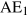. Equilibrium GDP rises by a larger amount from Y0 to Y1.Figure 6.8 shows that an upward shift in the AE function increases equilibrium income by a finite amount, but by a larger amount than the vertical rise in the AE line. This is because (c–m), the slope of AE, is less than unity, giving the AE line a lower slope than the line. Households increase their expenditure when incomes rise, but they increase expenditure by less than the rise in income. Equilibrium moves from Y0 to Y1. Equilibrium output rises more than the original rise in investment, , but does not rise without limit.

A fall in autonomous expenditure would have the opposite effect. AE would shift down and equilibrium income would decline by more than the fall in A.

The multiplier is a concept used to define the change in equilibrium output and income caused by a change in autonomous expenditure. If A is autonomous expenditure:(6.3)

Multiplier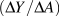: the ratio of the change in equilibrium income Y to the change in autonomous expenditure A that caused it.

We can also show the change in equilibrium output caused by a rise in autonomous investment expenditure using the earlier simple numerical example we used earlier. In Table 6.4 initial autonomous expenditure is 100, induced expenditure is 0.5Y and initial equilibrium is Y=200. Then autonomous expenditure increases, as in column (3) by 25 to a new level of 125. Induced expenditure is still 0.5Y. Aggregate expenditure in column (6) rises as a result of increase in both autonomous and induced expenditure.

Table 6.4 The effect of a rise in autonomous expenditure on equilibrium GDP
 Initial New Initial New GDP Autonomous Autonomous Induced Aggregate Aggregate (Y) Expenditure Expenditure Expenditure Expenditure Expenditure (A0=100) (A1=125) (c–m)Y=0.5Y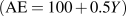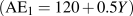(1) (2) (3) (4) (5) (6) 175 100 - 87.5 187.5 187.5 200 100 - 100 200 200 225 125 112.5 237.5 237.5 125 118.75 243.5 243.5 125 121.75 246.75 246.75 125 – 125 250 125 125 250 300 125 150 275

The initial increase in aggregate expenditure illustrated is from 200 to 237.5 made up of an increase in autonomous expenditure of 25 and in induced expenditure of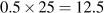. However GDP(Y) is only 225 and planned expenditure is accommodated by a decrease in inventories of 12.5. This unintended fall in inventories is an incentive to increase production to take advantage of higher than expected sales. The incentive exists until producers increase output and income to 250. Aggregate expenditure rises as income increases until equilibrium is reached at Y=250. At that point actual inventory investment meets producer plans.Figure 6.8 using the numerical values for GDP and aggregate expenditure in Table 6.4 and a line to show equilibrium Ye=200. Example Box 6.2 at the end of the chapter illustrates the multiplier effect of a change in autonomous expenditure on equilibrium income using simple algebra.

## The size of the multiplier

The multiplier is a number that tells us how much equilibrium output changes as a result of a change in autonomous expenditure. The multiplier is bigger than 1 because a change in autonomous expenditure changes income and sets off further changes in induced expenditure. The marginal propensity to spend on domestic output (cm) determines the induced expenditure.

In more general terms, because mpcmpm=cm is the slope of the AE function, we can write:(6.4)

In the example in Table 6.4 with a induced expenditure (cm)Y=0.5 wbich also the slope of AE the multiplier is: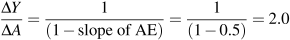But at a different time or in a different economy the marginal propensity to consume might be higher and the marginal propensity to import might be lower, for example let c1=0.85 and m1=0.10. Then induced expenditure on domestic output would be (c1m1)=(0.85–0.10)=0.75 as would the slope of. Now a change in autonomous expenditure raises income and causes a higher change in induced expenditure. As a result the multiplier for this economy would be:As a result, national incomes in economies with higher rates of induced expenditure on domestic output will fluctuate more; experience stronger business cycles, as a result of changes in autonomous expenditure like investment and exports.

In the basic model we have here, the slope of AE is (mpcmpm), but this simple formula for the multiplier will still be useful when we introduce the tax system in the government sector in Chapter 7.

This page titled 6.4: The multiplier - Changes in aggregate expenditure and equilibrium output is shared under a CC BY-NC-SA license and was authored, remixed, and/or curated by Douglas Curtis and Ian Irvine (Lyryx) .